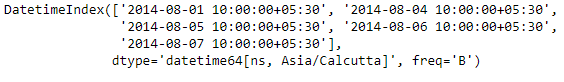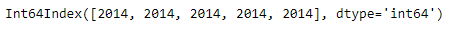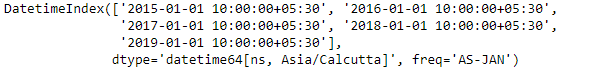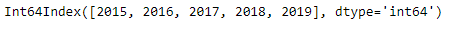# Python | Pandas DatetimeIndex.year

• Last Updated : 24 Dec, 2018

Python is a great language for doing data analysis, primarily because of the fantastic ecosystem of data-centric python packages. Pandas is one of those packages and makes importing and analyzing data much easier.

Pandas` DatetimeIndex.year` attribute outputs an Index object containing the value of years present in the Datetime object.

Syntax: DatetimeIndex.year

Return: Index containing years.

Example #1: Use `DatetimeIndex.year` attribute to find the years present in the DatetimeIndex.

 `# importing pandas as pd``import` `pandas as pd`` ` `# Create the DatetimeIndex``# Here the 'B' represents Business day frequency``didx ``=` `pd.DatetimeIndex(start ``=``'2014-08-01 10:00'``, freq ``=``'B'``, ``                            ``periods ``=` `5``, tz ``=``'Asia/Calcutta'``)`` ` `# Print the DatetimeIndex``print``(didx)`

Output :Now we want to find all the year values present in the DatetimeIndex object.

 `# find all the years in the object``didx.year`

Output :As we can see in the output, the function has returned an Index object containing the year value of each entry in the DatetimeIndex object.
&n bsp;
Example #2: Use `DatetimeIndex.year` attribute to find the years present in the DatetimeIndex.

 `# importing pandas as pd``import` `pandas as pd`` ` `# Create the DatetimeIndex``# Here the 'AS' represents Year start frequency``didx ``=` `pd.DatetimeIndex(start ``=``'2014-08-01 10:00'``, freq ``=``'AS'``, ``                             ``periods ``=` `5``, tz ``=``'Asia/Calcutta'``)`` ` `# Print the DatetimeIndex``print``(didx)`

Output :Now we want to find all the year values present in the DatetimeIndex object.

 `# find all the years in the object``didx.year`

Output :As we can see in the output, the function has returned an Index object containing the year value of each entry in the DatetimeIndex object.

My Personal Notes arrow_drop_up